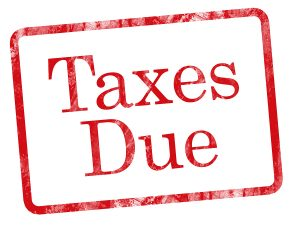## I have a Delaware corporation; how do I calculate my franchise tax?Delaware provides two ways to calculate the franchise tax. The minimum tax is \$175 (plus a \$25 report fee) and the maximum tax is \$200,000 plus the report fee. The calculations below are based upon the 2018 franchise tax rate for the tax due on or before May 1, 2019. The calculations are somewhat obtuse, but we have tried to simplify the calculations for you.

Corporations without par value stock, the authorized share method will always result in the lesser tax:

• 5,000 shares authorized or less (whether issued or not) pay the minimum tax of \$175.00
• 5,001 shares to 10,000 shares pay an addition \$250.00
• The tax is calculated on the number of shares authorized in the certificate of incorporation. If the company is authorized to issue 10 million shares but only 100 shares have been issued and are outstanding, the company will pay the tax on the 10 million authorized shares and not on the 100 shares issued and outstanding.
• You can see that a company that over authorizes will pay a penalty.
• Example 1, a corporation with 10,005 authorized shares pay \$335.00 (\$250  for the first 10,000 shares plus \$85 for the additional shares above 10,000 which is not prorated.)
• Example 2, a corporation with 100,000 no par shares plus \$85.00 [for each 10,000 additional shares without proration] times 9.

Assumed Par Value Method (of the “Alternative Method”) applicable to corporations with par value stock:

• Using this method requires the disclosure to the State of substantial information.
• You must provide:
• Number of all issued shares including treasury shares
• Total Gross assets reported on Schedule L to US Form 1120 relative to fiscal year ending the calendar year of the report. The state reserves the right to require that the company provide a copy of the Federal return to confirm the accuracy of the Assets reported to the State.
• The tax rate is \$400.00 per million or portion of a million dollars of par value. If the assumed par value is les than \$1,000,000, the tax is calculated by dividing the assumed par value capital by \$1,000,000 then multiplied by \$400.
• Example: a corporation has 1,000,000 shares of stock with a par value of \$1.00 and 250,000 share of stock with a par value of \$5.00, gross assets on its schedule L or \$1,000,000 with a total of only 485,000 shares outstanding.
• Calculation of tax:
• Divide the \$1,000,000 of total gross asset by 485,000 carried to 6 decimal places, \$2.061856 which is the “assumed par value per share”
• Multiply the assumed par value per share by the number of “authorized shares having a par value of less than the assumed par value, in this case the par value is \$1.00 and the assumed par value per share is \$2.061856, so you multiply times 1,000,000 authorized share of \$1.00, resulting in the sum of \$2,061,856.
• In this example the company also authorized as second series of  250,000 shares with a par value of \$5.000. Under the rules you multiply the number  of authorized shares with a par value greater the assumed par value by its par value, 250,000 x \$5.00=\$1,250,000.
• Add the two sums above, \$2,061,856 plus \$1,250,000= \$3,311,856, the “assumed par value capital”
• The tax is calculated by dividing the “assumed par value capital” rounded up to the next million dollars, if it is in excess of \$1,000,000, times \$400.00. In our example \$3,311,856 is rounded up to “4”. 4 is then multiplied by \$400.00 resulting in a tax of \$1,600.00
• The minimum assumed par value tax is \$400.00.
• As you can see, if the company stock has a par value per share of less than one cent, which is permissible, the assumed par value method can easily reduce the tax to the \$400 minimum tax, notwithstanding that the company may have millions of shares authorized and outstanding. This is an option for companies which wish to have large amounts of stock authorized and issued.
• Using the above example where the corporation is authorized with 1,000,000 shares with a par value of \$0.00001. Using the above example \$2.061856 is the “assumed par value per share”. That number is then multiplied by the “authorized shares” of 1,000,000 resulting in the sum of \$2,061,856, rounded up to ne next million is “3”, multiplied by \$400 yields a tax of \$1200.

The Delaware Secretary of State offers a Franchise tax calculator for the 2018 franchise tax due on or before May 1, 2019 https://corpfiles.delaware.gov/taxcalc_2018.xls